Home | | Structural Dynamics and Earthquake Engineering | Forced vibration (harmonic force) of single-degree-of-freedom systems

# Forced vibration (harmonic force) of single-degree-of-freedom systems

Abstract: In this chapter, forced vibration of single-degree-of-freedom (SDOF) systems (both undamped and under-damped) due to harmonic force is considered. Governing equations are derived and the displacement response is determined using WilsonŌĆÖs recurrence formula. Vibration excitation due to imbalance in rotating machines is discussed. Equations for transmissibility are derived for force and displacement isolation. The underlying principle of vibration-measuring instruments is illustrated.

Forced vibration (harmonic force) of single-degree-of-freedom systems in relation to structural dynamics during earthquakes

Abstract: In this chapter, forced vibration of single-degree-of-freedom (SDOF) systems (both undamped and under-damped) due to harmonic force is considered. Governing equations are derived and the displacement response is determined using WilsonŌĆÖs recurrence formula. Vibration excitation due to imbalance in rotating machines is discussed. Equations for transmissibility are derived for force and displacement isolation. The underlying principle of vibration-measuring instruments is illustrated.

Key words: resonance, transient, steady state, magnification factor, beating, transmissibility, seismometer, accelerometer.

Forced vibration without damping

In many important vibration problems encountered in engineering work, the exciting force is applied periodically during the motion. These are called forced vibrations. The most common periodic force is a harmonic force of time such as

P = P0 sin Žēt   ŌĆ”ŌĆ”ŌĆ”ŌĆ” 4.1

where P0 is a constant, Žē is the forcing frequency and t is the time. The motion is analysed using Fig. 4.1.The general solution of Eq. 4.2 (non-homogeneous second order differential equation) consists of two parts x = xc + xp where xc = complementary solution,and xp = particular solution. The complementary solution is obtained by setting right hand side as zero.The first two terms of free vibration are dependent only on properties m and k of the system and also on initial conditions. This is called transient vibration because, in a real system, it is damped out by friction.

The third term represents forced vibration and depends on the amplitude of applied force and forcing frequency Žē (or ╬▓ = Žē/Žēn). This is called steady state vibration since it is the motion of the system after a transient vibration is dissipated.The amplitude is ╬┤st/(1 ŌĆ' ╬▓ 2) and it becomes infinite when ╬▓ = 1. This condition is called resonance.

Of course the amplitude does not become infinity in practice because of damping or physical constraints but the condition is a dangerous one, causing fractures. We define magnification factor asand it is plotted as shown in Fig. 4.2. Several items are of particular interest in this diagram.

Example 4.1

A 1500 kg truck cab is assumed to be supported by four springs each with stiffness 120 kN/m. Determine the resonant frequency of the cab in unit of Hz and the amplitude of vibration if the displacement input of each accelerator is d = 0.05 sin 6t.

Solution

We know that

mx╦Ö╦Ö + kx = P0 sin Žē t

m = 1500 kg; k = 4 ├- 120 ├- 1000 = 480 ├- 1000

P0 = k╬┤ = 480 ├- 1000 ├- 0.05 = 24 000 N

Substituting in the above equation,

1500 ╦Ö╦Öx + 480 ├- 1000 x = P0 sin 6tEquation 4.12 is also written as

x = X sin (Žēnt + Žå) + ╬┤st sin (Žēt)/(1ŌĆ' ╬▓2)   ŌĆ”ŌĆ”ŌĆ”    ŌĆ”. 4.15

Three distinct types of motion are possible depending on whether the value of frequency ratio ╬▓ is less than, equal to or greater than 1.

If ╬▓ <1 then Žē < Žēn indicating that the natural frequency response (transient) is greater than forced response (steady state). The resulting motion is represented in Fig. 4.3a. The free vibration portion of the motion completes several cycles in the time required for one cycle of the forced response. The total motion (depicted by dotted line) exhibits a sinusoidal variation about a lower frequency base curve (represented by the solid line in Fig. 4.3a. In this case the forced response is greater than the equivalent static deflection ╬┤st.

When the frequency ratio ╬▓ > 1 then Žēn < Žē and the total motion is characterized as the forced response oscillating about the free vibration portion of the response as indicated in Fig. 4.3b. Also if ╬▓ > 2 , the amplitude of the forced response will be less than the equivalent static deflection ╬┤st.Example 4.2

The undamped springŌĆ'mass system has a mass of 4.5 kg and a spring stiffness of 3500 N/m. It is excited by a harmonic force having an amplitude F0 =100 N and an excitation frequency of Žē =10 rad/s. The initial conditions are x(0) = 0.015 m and v(0) = 0.15 m/s. Determine (a) the frequency ratio (b) the amplitude of the forced response (c) the displacement of the mass at time t = 2 s. and (d) the velocity of mass at time t = 4 s. Draw the forced response and total response curves. If the excitation frequency is 40 r/s determine how the forced response and total response curves change.

Solution

The natural frequency of the system is calculated asUsing the initial conditions x(0) = 0.015 m and v(0) = 0.15 m/s we get

C 2  = 0.015; C1  = 0.004 20

Substituting these values in displacement equation and t = 2 s, we get x (t = 2) = 0.037 79m and v(t = 2) = 0.2270 m/s. The harmonic response of an undamped single-degree-of-freedom (SDOF) system to harmonic excitation for this problem is shown in Fig. 4.3a. When the excitation frequency is 40 rad/s then ╬▓ = 1.434; the harmonic response is shown in Fig. 4.3b.

Beating phenomenon

Two very important phenomena occur when the frequency of the forcing function Žē approaches natural circular frequency of the system Žēn or when ╬▓ Ōå' 1. First consider Žē and Žēn are nearly the same or Žēn is slightly greater than Žē.Žē is much larger than ╬Ą in the term sin (╬Ąt) and oscillates with much larger period than cos (Žēt) does. The resulting motion, illustrated in Fig. 4.4, is a rapid oscillation with slowly varying amplitude and referred to as beat. Sometimes the two sinusoids add to each other, and at other times they cancel each other out, resulting in a beating phenomenon.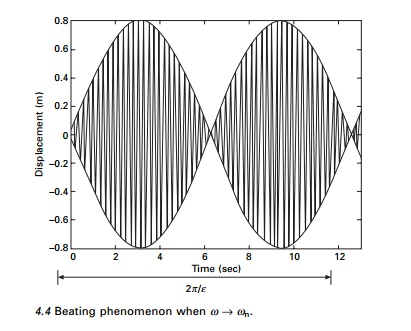Example 4.3

An undamped system is harmonically forced resulting in a beating condition. The natural and excited frequencies are 1500 cycles/min and 1450 cycles/ min respectively. Determine (a) beat period (b) number of oscillations in each beat and (c) the maximum amplitude of oscillation if W = 50 kN and the amplitude of steady state force is 25 kN.Resonance

When the excited frequency is exactly equal to the natural circular frequency we get a condition known as resonance. At this point ╬▓ = 1 and the amplitude of vibration increases without bound. For this the solution given in Eq. 4.16 is no longer valid. The particular solution for the equation Eq. 4.5 now isThe first term is predominant and the amplitude varies linearly with respect to time as shown in Fig. 4.5.

Example 4.4

An SDOF system has a total weight of 5 kN and a spring stiffness of 360kN/ m. The system is excited at resonance by a harmonic force of 3 kN. Determine the displacement amplitude of the forced response after (a) 1.25 cycles and

(b) 10.25 cycles.

Solution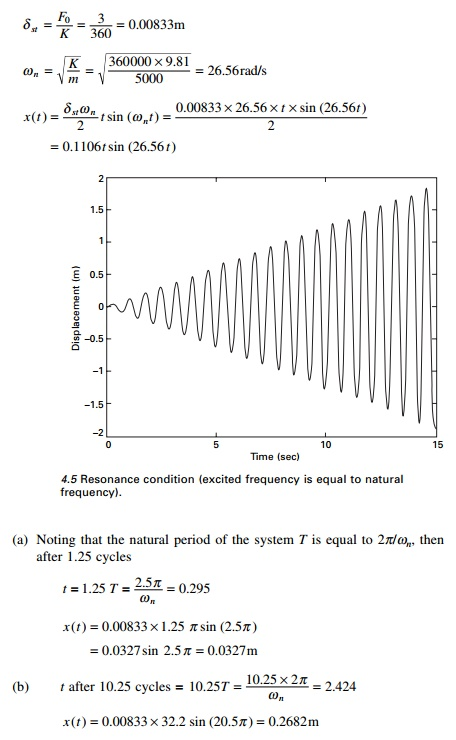Equation 4.19 indicates that for a system operating at resonance, the amplitude of the forced response increases linearly with time by ŽĆ╬┤st per cycle as indicated in Fig. 4.5. Theoretically, the amplitude will eventually approach infinity. In reality, however, the system will break down once the amplitude becomes intolerably large for the structure. Fortunately, since the steady state amplitude varies directly with time, the system would have to operate at resonance for an extended period before the amplitude becomes destructively large. Therefore, it is acceptable for a system or a machine in route to its operating frequency to quickly pass through the resonance amplitude.

Forced vibration with damping

Consider a forced vibration of the under-damped system shown in Fig. 4.6. The dynamic equilibrium equation is written as,

Equation 4.21 is a second order non-homogeneous equation and it has both a complementary solution xc and a particular solution xP . xc is same as that for free vibration of an under-damped system.and the plot of MF is shown in Fig. 4.7.

The dramatic increase in MF near the natural frequency Žēn is called resonance and Žē is called the resonance frequency. The following items are of interest in the diagram.

┬Ģ                Static loading: Žē = 0;╬┤/P0 /k = 1. It is independent of damping.

ŌĆó       Resonance: Žē = Žēn the amplitude is magnified substantially when the coefficient of viscous damping Žü is low.

ŌĆó       High-frequency excitation: Žē >> Žēn; ╬┤/╬┤st 0 the mass is essentially stationary because of its inertia of any damping of motion.

ŌĆó       Large coefficient of viscous damping: The amplitude of the vibration is reduced at all values of (Žē/Žēn) as the coefficient of viscous damping Žü is increased in a particular system.

Recurrence formula of Wilson

The recurrence formula is derived in a similar way to that of free vibration of an under-damped system. The displacement is given in terms of Eq. 4.30. The displacement x is written as(where the functions C and S are defined in Chapter 3) and co = cos (Žēt); si = sin (Žēt) where Žē is the excited frequency. Differentiating with respect to time t we get velocity expression asThe constants D and E are given in Eq. 4.25e and f respectively.

For the initial conditions of displacement and velocity the displacement at any time is given asApart from the last two terms other terms belong to transient part and the last two terms to steady state. Figure 4.8 shows the transient, steady state and total response for forced vibration of under-damped SDOF system.Program 4.2: MATLAB program for finding response due to harmonic force

clc close all

%****************************************************

%give mass of the system m=6;

%give stiffness of the system k=8;

wn=sqrt(k/m);

%give damping coefficient c1=2;

%give initial conditions - displacement and velocity u(1)=.25;

udot(1)=.5;

%give magnitude of harmonic force

f=4;

%give excited frequency of the force w=1;

%****************************************************

beta=w/wn;

cc=2*sqrt(k*m);

rho=c1/cc; wd=wn*sqrt(1-rho^2); wba=rho*wn; rhoba=rho/sqrt(1-rho^2); b0=2.0*rho*wn; b1=wd^2-wn^2; b2=2.0*wba*wd; dt=0.02;

t(1)=0;

for i=2:1500 t(i)=(i-1)*dt;

s=exp(-rho*wn*t(i))*sin(wd*t(i)); c=exp(-rho*wn*t(i))*cos(wd*t(i)); sdot=-wba*s+wd*c; cdot=-wba*c-wd*s; sddot=-b1*s-b2*c; cddot=-b1*c+b2*s; a1=c+rhoba*s;

a2=s/wd; d=-2.0*f*(rho*beta)/(k*(1-beta^2)^2+(2*rho*beta)^2);

e=f*(1-beta^2)/(k*(1-beta^2)^2+(2*rho*beta)^2); u(i)=a1*u(1)+a2*udot(1)-d*(c+wba*s/wd)-e*w*s/wd; v(i)=d*(cos(w*t(i)))+e*sin(w*t(i));

x(i)=u(i)+v(i); end

figure(1);

plot(t,x,ŌĆśkŌĆÖ); xlabel(ŌĆś timeŌĆÖ);

ylabel(ŌĆś displacement ŌĆÖ); title(ŌĆś displacement - timeŌĆÖ);

Vector relationship in forced vibration

For small values of Žē/Žēn << 1, both inertia and damping forces are small, which results in a small phase angle Žł. The magnitude of the impressed force is then nearly equal to the spring force as shown in Fig. 4.9a. For Žē/Žēn = 1, the phase angle is 90┬ o and the force diagram appears as in Fig. 4.9b. The inertia force, which is now larger, is balanced by the spring force; whereas the impressed force overcomes the damping force. The amplitude at resonance can be found from Eq. 4.29a. At large values of Žē/Žēn > 1 the phase angle approaches 180┬ o and the impressed force is expended almost entirely in overcoming the large inertia force as shown in Fig. 4.9c

Example 4.5

An SDOF system shown in Fig. 4.10 is modelled as 3000kg mass on a spring stiffness k = 400 kN/m. The system has a damping factor of c/cc= 0.4. Assume that the spring is attached to the base whose vertical displacement are defined by d = 0.04 sin 6t. Write the equation of motion of m for steadyvibration. Determine the magnification factor of the amplitude of vibration, the amplitude A and phase angleŽł.Example 4.6

A weight attached to a spring of stiffness 530 N/m and undergoes viscous damping and the weight was displaced and released as shown in Fig. 4.11.The period of vibration was found to be 1.8 seconds. The ratio of consecutive amplitudes was found to be 4.2/1. Determine the amplitude and phase angle when a force of 200 cos 3t acts on the system.

Solution

Given k = 530 N/m; t = 1.8 seconds; (xn+1/xn) = 4.2

Logarithmic decrement = loge 4.2 = 1.435Hence XP = 0.779 sin (3t ŌĆ' Žł) is a steady state.

Example 4.7

The spring of an automobile trailer shown in Fig. 4.12 is compressed under its weight by 100mm. Find the critical speed when the tractor is travelling over a road with a profile approximated by a sine wave of amplitude 75mm and a wavelength of 16m. What will be the amplitude of vibration at 64 km/h? Neglect damping.

Solution

Profile of the road = y = 0.075 sin (2ŽĆs/16)

The equation of motion mx╦Ö╦Ö + k ( x Ōł' y) = 0 or mx╦Ö╦Ö + kx = ky

╦Ö╦Öx + Žē n2 ╬┤x = Žē n2 y

s = distance covered = vtRotating imbalance

Imbalance in rotating machines is a common source of vibration excitation. Let us consider a springŌĆ'mass system constrained to move in the vertical direction and excited by a rotating machine that is unbalanced as shown in Fig. 4.13. The imbalance is represented by an eccentric mass m with eccentricity e rotating with angular velocityŽē.

Let x be the displacement of the non-rotating mass (MŌĆ'm) from the static equilibrium position, and the displacement of m is

x + e sin Žēt

The equation of motion is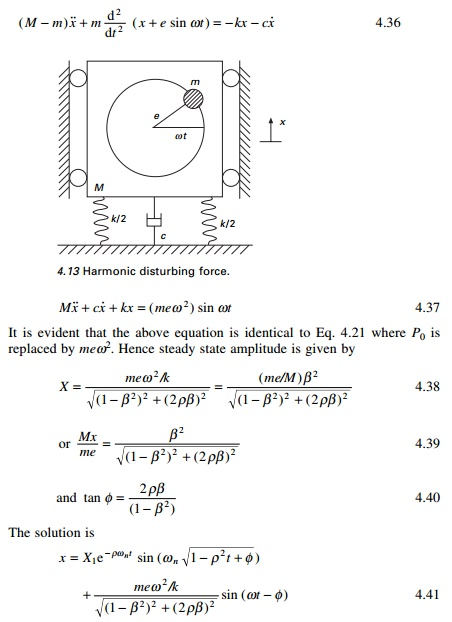MX/me versus frequency is plotted in Fig. 4.14 for various damping ratios. The phase angle with respect to frequency ratio for various damping values is the same as in Fig. 4.7.

Example 4.8

A machine part having a mass of 2.5kg vibrates in a viscous medium. A harmonic exciting force of 30N acts on the part and causes resonant amplitude of 14 mm with a period of 0.22s. Find the damping coefficient. If the frequency of the exciting force is changed to 4 Hz, also determine the increase in the amplitude of the forced vibration upon the removal of the damper.

SolutionExample 4.9

A single cylinder vertical diesel engine has a mass of 400kg and is mounted on a steel chassis frame. The static deflection owing to the weight of the chassis is 2.4mm. The reciprocating mass of the engine is 18kg and the stroke of the engine is 160 mm. A dashpot with a damping coefficient of 2N/mm/s is also used to dampen the vibration. In the steady state of vibration, determine (a) the amplitude of the vibration if the driving shaft rotates at 500 rpm and (b) the speed of the driving shaft when the resonance occurs.

Solution

Given m = 400 kg; N = 500rpm; c = 2000 N/m/s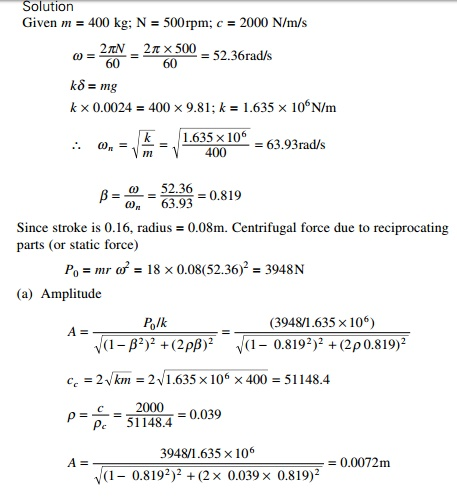Example 4.10

A body having a mass of 15kg is suspended from a spring which deflects 12mm under the weight of the mass. Determine the frequency of free vibration and also the viscous damping force needed to make the motion periodic or a speed of 1mm/s.

When dampened to this extent, a disturbing force having a maximum value of 100N and vibrating at 6Hz is made to act on the body. Determine the amplitude of ultimate motion.

Solution

Given 15kg; ╬┤st = 12mm; P0 = 100N; f = 6HzThe motion becomes a periodic when the damped frequency is zero or when it is critically damped, i.e. Žü = 1 and Žē = Žēn = 2ŽĆf = 2ŽĆ ├- 4.55 = 28.59 rad/sTransmissibility (force isolation)

Consider the springŌĆ'massŌĆ'damper system subjected to harmonic force shown in Fig. 4.15 which is generated by machines and engines. These vibrations are often unavoidable; however, their effect on a dynamical system can be reduced significantly by properly designed springs and damper system known as isolators.

We know that the displacement response is given byProgram 4.3: MATLAB program to compute MF,

MX/me and TR

for i=1:6 rho=i*0.2; z(i)=rho; for j=1:61

x(j)=(j-1)*0.1;

% take y according to 1 - harmonic force % 2 - machine with imbalance

%                 3 - transmissibility

% 1- for harmonic force

% y(j,i)=1/(sqrt((1-x(j)^2)^2+(2*rho*x(j))^2));

% 2- when the machine running with imbalance mass with speed omega

%y(j,i)=x(j)^2/(sqrt((1-x(j)^2)^2+(2*rho*x(j))^2));

%3- Transmissibility

y(j,i)=sqrt(1+(2.0*rho*x(j))^2)/(sqrt((1-x(j)^2)^2+(2*rho*x(j))^2));

end

end

for i=1:61 z1(i)=y(i,1) z2(i)=y(i,2) z3(i)=y(i,3) z4(i)=y(i,4) z5(i)=y(i,5) z6(i)=y(i,6)

end

%1- ylabel MF

%2- ylabel MX/me

3- ylabel TR

figure(1)

plot(x,z1,x,z2,x,z3,x,z4,x,z5,x,z6) xlabel(ŌĆś timeŌĆÖ)

ylabel(ŌĆś TR factorŌĆÖ) gtext(ŌĆś rho=0.2ŌĆÖ) gtext(ŌĆś rho=0.4ŌĆÖ) gtext(ŌĆś rho=0.6ŌĆÖ); gtext(ŌĆśrho=0.8ŌĆÖ) gtext(ŌĆśrho=1.0ŌĆÖ) gtext(ŌĆś rho=1.2ŌĆÖ) figure(2) surf(z,x,y); zlabel(ŌĆśTRŌĆÖ); xlabel(ŌĆś zetaŌĆÖ) ylabel(ŌĆś betaŌĆÖ)

Figures 4.16 and 4.17 show the variation of TR for various values of frequency ratios for different values of damping.

Effectiveness of foundation

For the design of a vibration isolation system, it is convenient to express behaviour of the system in terms of isolation effectiveness rather than transmissibility effectiveness. An isolation system (see Fig. 4.15) is effective only if ╬▓ > 1.414 and since damping is undesirable in that range, it is evidentthat isolated monitoring should have very little damping. When Žü = 0 and ╬▓ > 1.414 TR = 1/(╬▓2 ŌĆ' 1). Then in that case effectiveness of the foundation is given by (1ŌĆ'TR).

Simplifying we get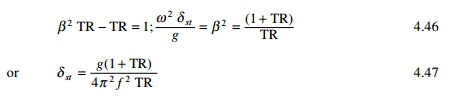Displacement isolation

Consider the spring mass damper system shown in Fig. 4.18 in which the support moves by xs. The equation of motion is written as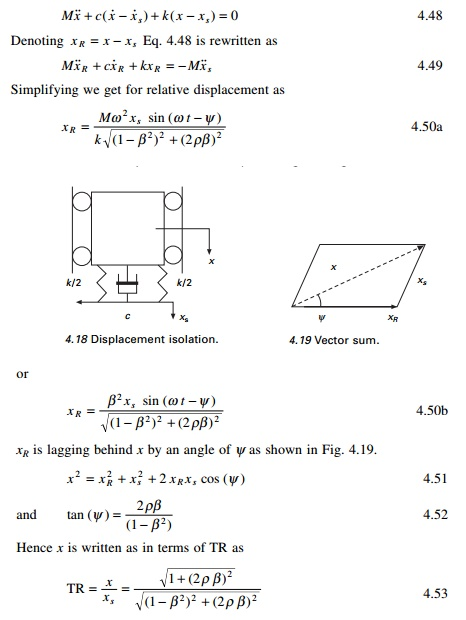Hence transmissibility of force and displacement are the same.

Vibration-measuring instruments

ŌĆó       Seismometer: This works for large values of ╬▓ or a low value of natural frequency. Hence a seismometer is a low natural frequency instrument. When ╬▓ becomes infinity, the relative displacement xR becomes equal to xs (see Eq. 4.50a). The mass M then remains stationary while the supporting case moves with the vibrating body. A large seismometer is required.

This instrument will have natural frequency of 2ŌĆ'5 Hz and a useful range of 10ŌĆ'500Hz.

Accelerometer: These instruments are of smaller size with high sensitivity. Using the instrument acceleration is obtained and velocity and displacement are obtained by integration. Examining Eq. 4.50a when ╬▓ Ōå' 0Accelerometers are high-frequency instruments and the useful range for ╬▓ is from zero to 0.4. Those used for earthquake measurements have a natural frequency of 20 Hz, which allows ground motion of frequency less than 8Hz to be reproduced. This is shown in Fig. 4.20.

How to evaluate damping in SDOF

1  Amplitude decrement in free vibration

We have seen in last Session (Pages) that logarithmic decrement is written asBy using a minimal instrumentation, one can find the damping coefficient by measuring amplitudes at m and m + n cycles.

2  Measuring resonant amplitude

Harmonic excitation is applied to the structure by applying harmonic load F0 sin (Žēt). A frequencyŌĆ'response curve for a damped structure is shown in Fig. 4.7. The dynamic magnification factor (DMF) is given by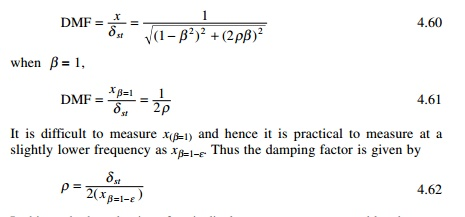In this method, evaluation of static displacement may pose a problem because many type of loading systems cannot be operated at zero frequency.

3  Bandwidth methods (see Fig. 4.21)

To determine the damping factor by this method determine the frequency ratio ╬▓ for whichExample 4.11

Data collected from a frequency response test of a structure were plotted to construct a response curve similar to the one shown for DMF vs. frequency ratio. From the plot it was determined that DMFmax was 1.35 and DMF at half power points was 0.95. The response ratios corresponding to half power points are 0.91 and 1.05 respectively. Estimate the amount of damping in the system.Response to ground acceleration

Referring to Fig. 4.15 when the base moves by xg the equation of motion is written asExample 4.12

A sensitive instrument with weight 500N is to be installed at a location where vertical acceleration is 0.1g and at frequency = 10Hz. This instrument is mounted on a rubber pad of stiffness 12800 N/m and damping such that the damping factor is 0.1.

(a)  What acceleration is transmitted to the instrument?

If the instrument can tolerate only an acceleration of 0.005 suggest a solution assuming that the same rubber pad is used.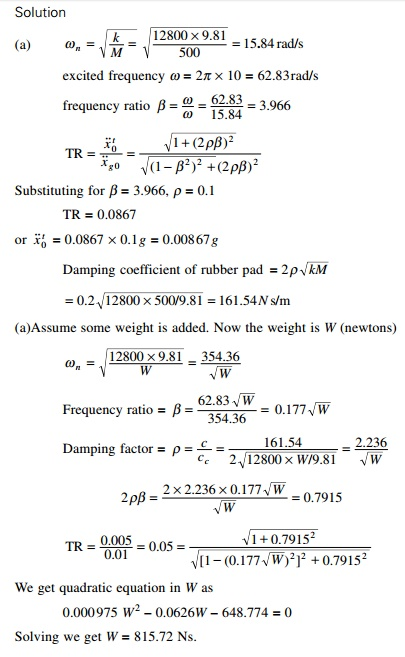Study Material, Lecturing Notes, Assignment, Reference, Wiki description explanation, brief detail
Civil : Structural dynamics of earthquake engineering : Forced vibration (harmonic force) of single-degree-of-freedom systems |